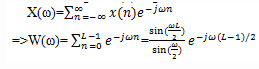# Test: DFT Signal Analysis

## 10 Questions MCQ Test Digital Signal Processing | Test: DFT Signal Analysis

Description
Attempt Test: DFT Signal Analysis | 10 questions in 10 minutes | Mock test for Electrical Engineering (EE) preparation | Free important questions MCQ to study Digital Signal Processing for Electrical Engineering (EE) Exam | Download free PDF with solutions
QUESTION: 1

### If the signal to be analyzed is an analog signal, we would pass it through an anti-aliasing filter with B as the bandwidth of the filtered signal and then the signal is sampled at a rate:

Solution:

Explanation: The filtered signal is sampled at a rate of Fs≥ 2B, where B is the bandwidth of the filtered signal to prevent aliasing.

QUESTION: 2

### What is the highest frequency that is contained in the sampled signal?

Solution:

Explanation: We know that, after passing the signal through anti-aliasing filter, the filtered signal is sampled at a rate of Fs≥ 2B=>B≤ Fs/2.Thus the maximum frequency of the sampled signal is Fs/2.

QUESTION: 3

### The finite observation interval for the signal places a limit on the frequency resolution.

Solution:

Explanation: After sampling the signal, we limit the duration of the signal to the time interval T0=LT, where L is the number of samples and T is the sample interval. So, it limits our ability to distinguish two frequency components that are separated by less than 1/T0=1/LT in frequency. So, the finite observation interval for the signal places a limit on the frequency resolution.

QUESTION: 4

If {x(n)} is the signal to be analyzed, limiting the duration of the sequence to L samples, in the interval 0≤ n≤ L-1, is equivalent to multiplying {x(n)} by:

Solution:

Explanation: The equation of the rectangular window w(n) is given as
w(n)= 1, 0≤ n≤ L-1
=0, otherwise
Thus, we can limit the duration of the signal x(n) to L samples by multiplying it with a rectangular window of length L.

QUESTION: 5

What is the Fourier transform of rectangular window of length L?

Solution:

Explanation: We know that the equation for the rectangular window w(n) is given as
w(n)= 1, 0≤ n≤ L-1
=0, otherwise
We know that the Fourier transform of a signal x(n) is given asQUESTION: 6

If x(n)=cosω0n and W(ω) is the Fourier transform of the rectangular signal w(n), then what is the Fourier transform of the signal x(n).w(n)?

Solution:

Explanation: According to the exponential properties of Fourier transform, we get
Fourier transform of x(n).w(n)= 1/2[W(ω-ω0)+ W(ω+ω0)]

QUESTION: 7

The characteristic of windowing the signal called “Leakage” is the power that is leaked out into the entire frequency range.

Solution:

Explanation: We note that the windowed spectrum X ̂(ω) is not localized to a single frequency, but instead it is spread out over the whole frequency range. Thus the power of the original signal sequence x(n) that was concentrated at a single frequency has been spread by the window into the entire frequency range. We say that the power has been leaked out into the entire frequency range and this phenomenon is called as “Leakage”.

QUESTION: 8

Which of the following is the advantage of Hanning window over rectangular window?

Solution:

Explanation: The Hanning window has less side lobes and the leakage is less in this windowing technique.

QUESTION: 9

Which of the following is the disadvantage of Hanning window over rectangular window?

Solution:

Explanation: In the magnitude response of the signal windowed using Hanning window, the width of the main lobe is more which is the disadvantage of this technique over rectangular windowing technique.

QUESTION: 10

The condition with less number of samples L should be avoided.

Solution:

Explanation: When the number of samples L is small, the window spectrum masks the signal spectrum and, consequently , the DFT of the data reflects the spectral characteristics of the window function. So, this situation should be avoided.Use Code STAYHOME200 and get INR 200 additional OFF Use Coupon Code